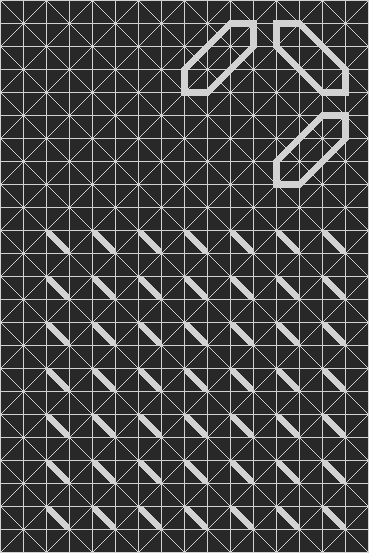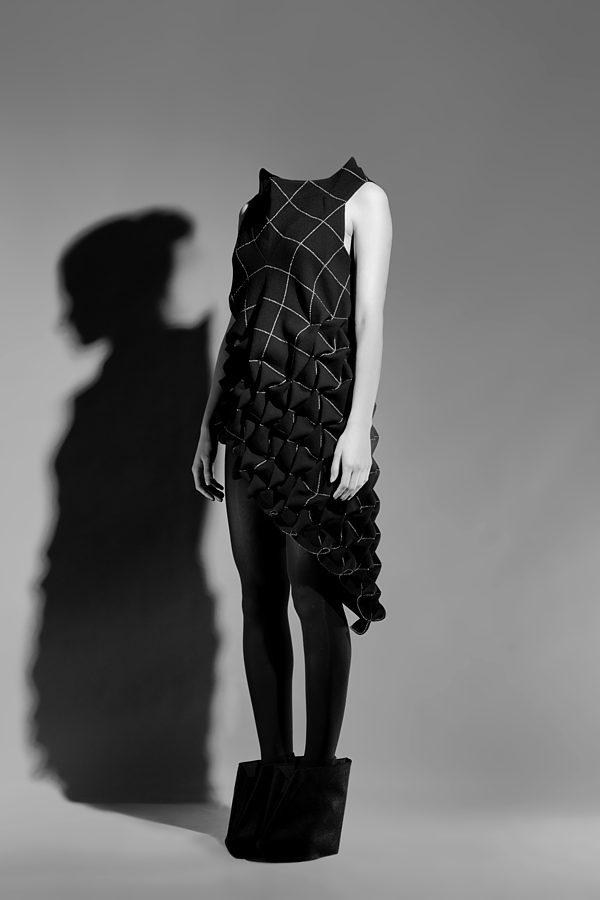## 18 September 2012

### THE ITERATION by Lisa ShahnoTHE ITERATION, (collection) 2012 by Lisa Shahno_
"The collection is inspired by the Fractal Cosmology theory which maintains the structure of the universe to be of the fractal nature and the universe itself to be infinite in any direction. A fractal is a kind of geometric shape which can be divided into parts, each at least approximately a reduced-size semblance of the whole, or a self-similar shape. According to the theory, there is a hierarchical organization (or nesting) of matter - from the elementary particles to the clusters off galaxies, with three main levels: atomic, astral and galactic. So the central issue of Fractal Cosmology is that the universe may consist of infinite number of levels which are similar to each other but different in scale - thereby there could be no "smallest" nor "largest" scales - the whole observable universe can be enclosed inside a molecule of some larger-scale universe and at the same time an atom may contain another world with its own galaxies, stars and inhabited planets..."  See more;

"Models of the collection represent a variety of matter levels in the universe. All pieces' patterns are composed of the one repeating basic element - the square divided by diagonals - an elementary particle, but each model has a different scale and number of particles involved." - Lisa Shahno.

Grid I (Fractal dimension = 1)
Catwalk speed ( V ) = 15 cm/secondGrid II (Fractal dimension = 0,5)
V = 30 cm/second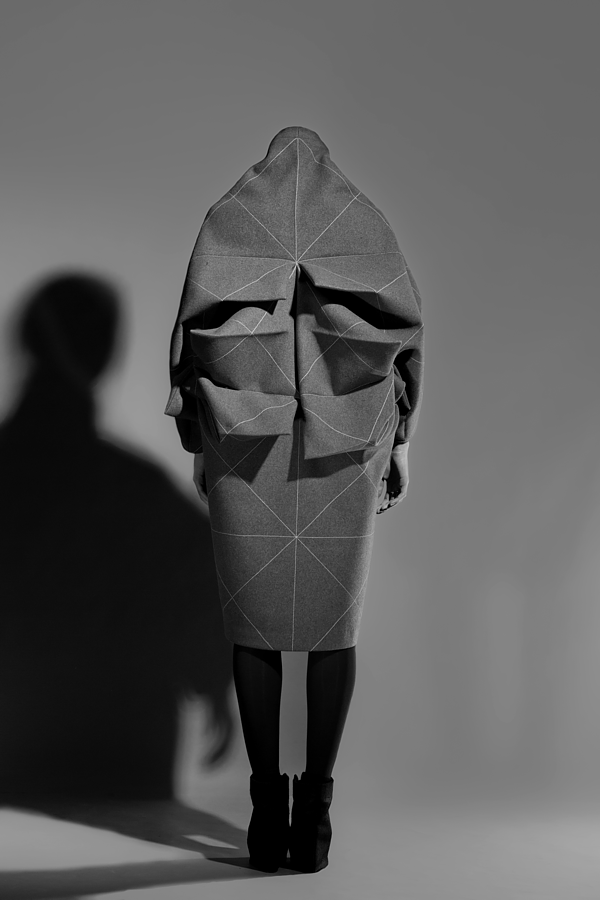Grid III (Fractal dimension = 0,33)
V = 50 cm/second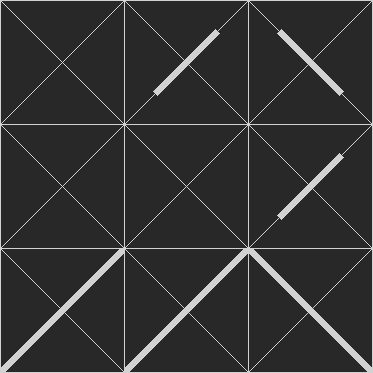Grid IIII (Fractal dimension = 0,14)
V = 100 cm/second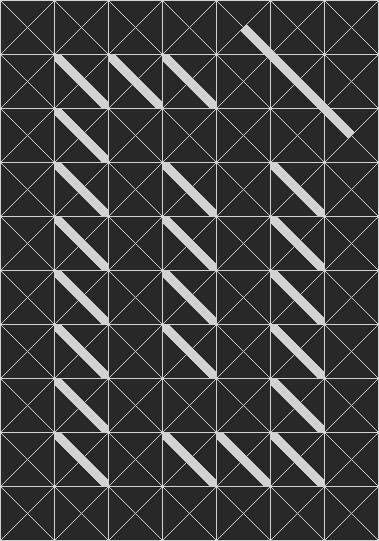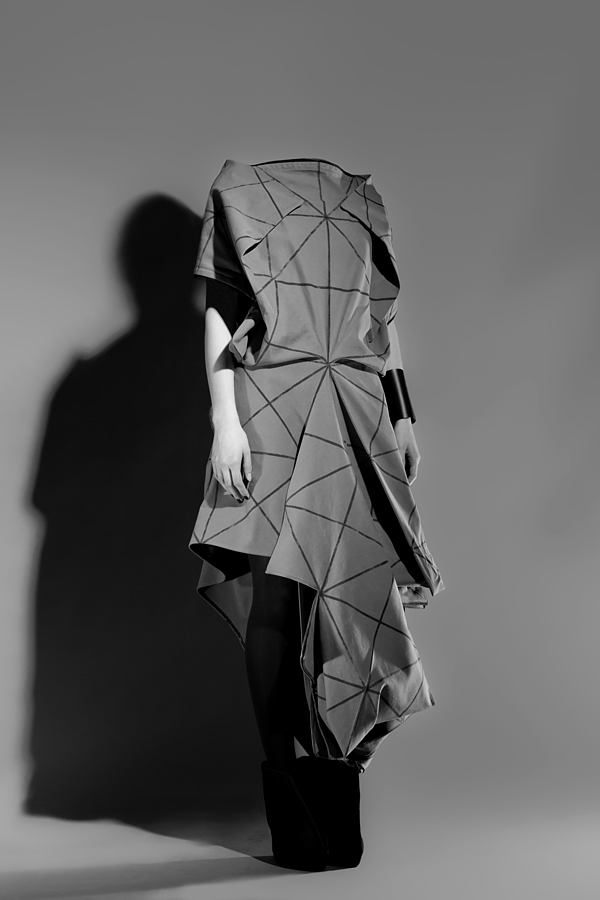Grid V (Fractal dimension = 0,14)
V = 100 cm/second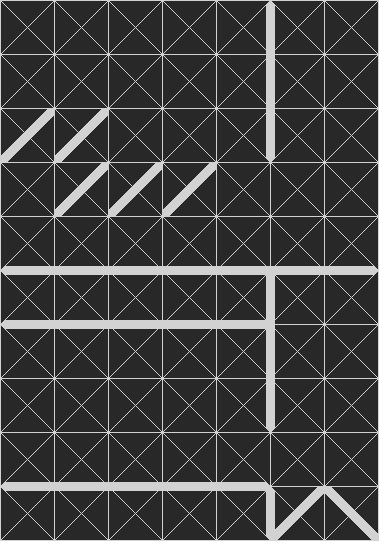Grid VI (Fractal dimension = 0,12)
V = 150 cm/second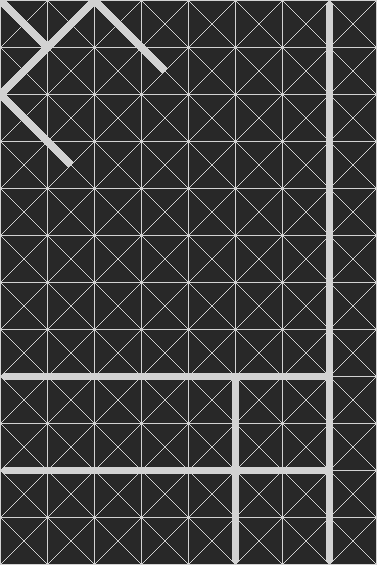Grid VII (Fractal dimension = 0,12)
V = 150 cm/second